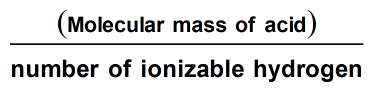Request a Tool

Equivalent Mass Calculator

The equivalent mass of the acid is equal to the number of grams of an acid per mole

Output

Equivalent mass of an acid (EM)
0

FormulaDefination / Uses

The number of parts by mass of an acid that contains 1.008 parts by mass of replaceable hydrogen is known as its equivalent mass. The amount of gram of acid per mole of accessible H+ ion is equal to the acid's equivalent mass:

One mole of acid ——- one equal quantity of acid I H+

The molar mass and equivalent mass are always related by simple formulae, but we need to know the acid's molecular formula to calculate the molar mass from the equivalent value. Use our calculator to get exact equivalant mass of an acid. The equivalent weight of acid is calculated using this Acid Weight Calculator. Consider the following scenario:
C2H6 has a molecular mass of roughly 30 ((2 x 12) + (6 x 1)). As a result, the molecule is about 2.5 times heavier than the 12C atom, or about the same mass as the NO atom, which has a molecular mass of 30 or (14+16). Use the upper given formula for manual calculations. No sign-up, registration OR captcha is required to use this tool.